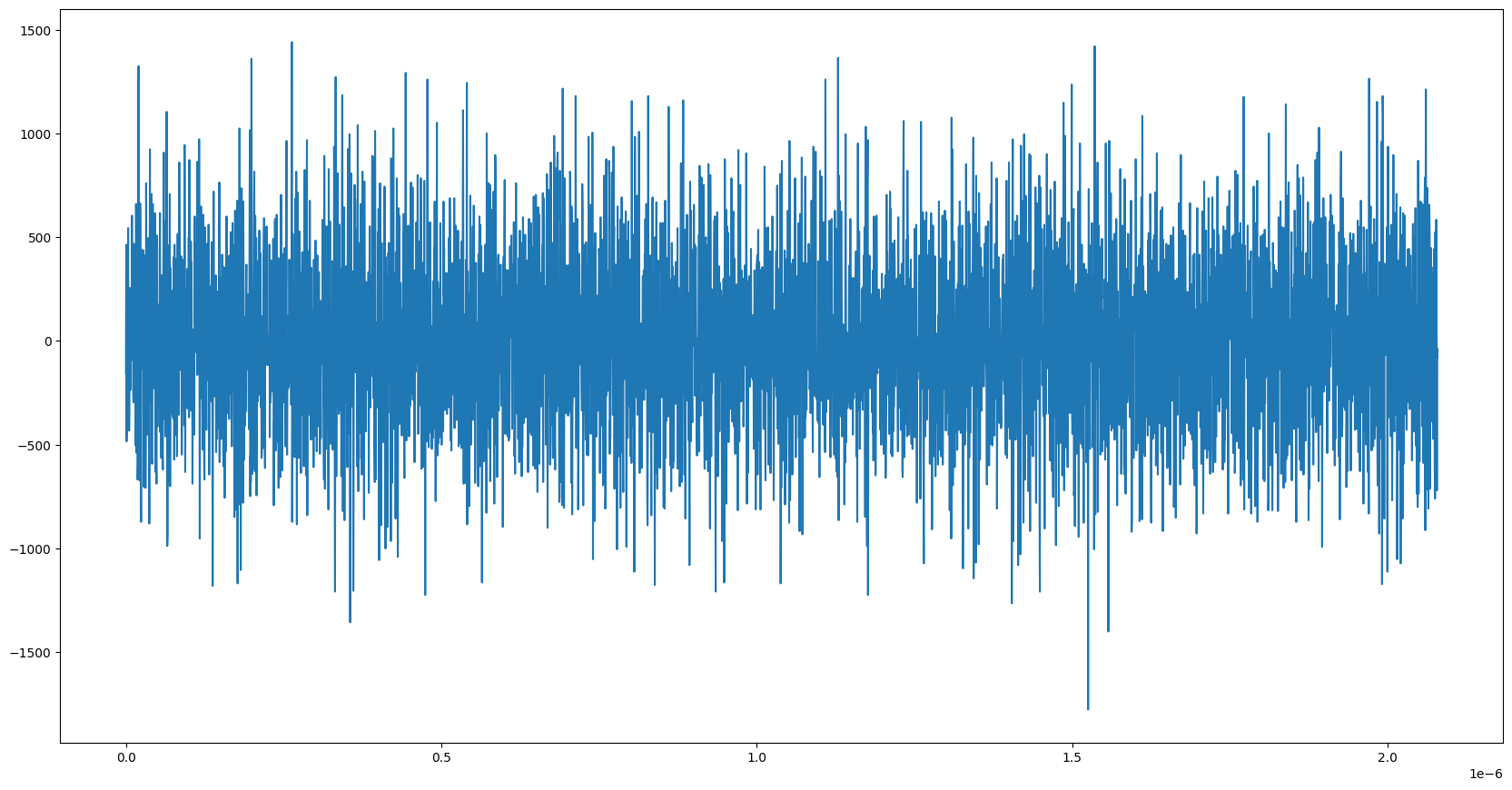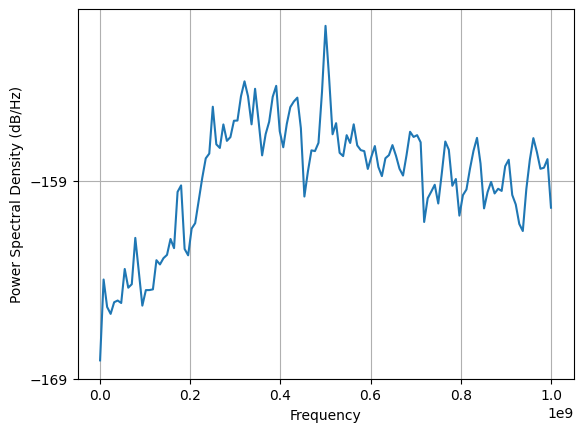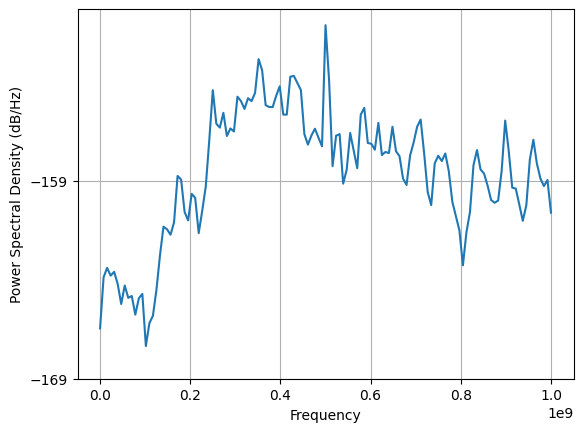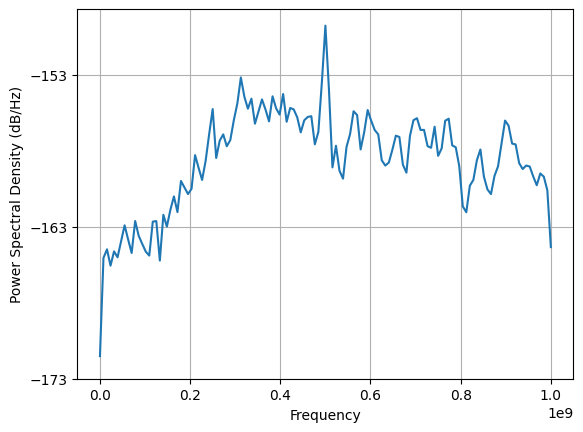# Accessing raw measurement data with RAW-CAPTURE¶

In this we are going to show how to access “raw” measurement data with Quilt.

:

from pyquil import Program, get_qc

qc = get_qc('Aspen-M-3')

:

cals = qc.compiler.get_calibration_program()


## Peeking at a MEASURE calibration¶

We first take a peek at how a measurement operation is specified. We can do this by looking at the corresponding calibration. Below we consider the calibration for MEASURE 0.

:

from pyquil.quilatom import Qubit, Frame
from pyquil.quilbase import Pulse, Capture, DefMeasureCalibration

qubit = Qubit(0)

measure_defn = cals.measure_calibrations

print(measure_defn)

DEFCAL MEASURE 0 addr:
FENCE 0
DECLARE q0_unclassified REAL
PULSE 0 "rf_f12" drag_gaussian(alpha: -1.394451807996698, anh: -190000000, detuning: 0, duration: 4e-8, fwhm: 1e-8, phase: 0, scale: 0.28637132443339525, t0: 2e-8)
NONBLOCKING PULSE 0 "ro_tx" flat(detuning: 0, duration: 2.08e-6, iq: 1, phase: 0, scale: 0.158489319246111)
NONBLOCKING CAPTURE 0 "ro_rx" boxcar_kernel(detuning: 0, duration: 2.08e-6, phase: -0.12799541944924012, scale: 1) q0_unclassified
PRAGMA FILTER-NODE q0_unclassified "{'module':'lodgepole.filters.io','filter_type':'DataBuffer','source':'q0_ro_rx/filter','publish':true,'params':{},'_type':'FilterNode'}"
PRAGMA FILTER-NODE q0_classified "{'module':'lodgepole.filters.classifiers','filter_type':'SingleQLinear','source':'q0_ro_rx/filter','publish':false,'params':{'a':[1.0,0.0],'threshold':0.00044068585795624876},'_type':'FilterNode'}"
PRAGMA FILTER-NODE q0 "{'module':'lodgepole.filters.io','filter_type':'DataBuffer','source':'q0_classified','publish':true,'params':{},'_type':'FilterNode'}"
PULSE 0 "rf_f12" drag_gaussian(alpha: -1.394451807996698, anh: -190000000, detuning: 0, duration: 4e-8, fwhm: 1e-8, phase: 0, scale: 0.28637132443339525, t0: 2e-8)
FENCE 0



There are a few things note about the above:

1. The basic structure of MEASURE 0 addr is to apply a pulse on the "ro_tx" frame, and then perform a capture on the corresponding "ro_rx" frame.

2. Although the user may perform MEASURE 0 ro, the memory location required for this is a bit. Under the hood, CAPTURE writes a complex IQ value to the REAL region q0_unclassified.

3. The wrangling in order to map from q0_unclassified to addr is controlled through PRAGMA operations. These are important for downstream processing and execution of the Quil program. Tamper with them at your own risk!

## RAW-CAPTURE experiments¶

The value stored in q0_unclassified has already been processed on hardware: in particular, it is produced by demodulating a passband signal and then integrating against the CAPTURE waveform. What RAW-CAPTURE does is give you, the user, access to the raw values of that passband signal. In the following, execute programs with RAW-CAPTURE, and plot their results.

Before we begin, it will be useful to get some data associated with the above MEASURE calibration. In particular, the PULSE and CAPTURE operations, as well as the frame definition for 0 "ro_rx".

:

pulse = next(i for i in measure_defn.instrs if isinstance(i, Pulse))
print(pulse, "\n")
capture = next(i for i in measure_defn.instrs if isinstance(i, Capture))
print(capture, "\n")
frame = Frame([qubit], "ro_rx")
frame_defn = cals.frames[frame]
print(frame_defn)

PULSE 0 "rf_f12" drag_gaussian(alpha: -1.394451807996698, anh: -190000000, detuning: 0, duration: 4e-8, fwhm: 1e-8, phase: 0, scale: 0.28637132443339525, t0: 2e-8)

NONBLOCKING CAPTURE 0 "ro_rx" boxcar_kernel(detuning: 0, duration: 2.08e-6, phase: -0.12799541944924012, scale: 1) q0_unclassified

DEFFRAME 0 "ro_rx":
DIRECTION: "rx"
SAMPLE-RATE: 2000000000
INITIAL-FREQUENCY: 7359200001.83969
HARDWARE-OBJECT: "q0_ro_rx"


### An almost-trivial example¶

First, let’s just run a RAW-CAPTURE instruction. We will apply this to the above CAPTURE frame, i.e. 0 "ro_rx", and for the same duration the CAPTURE. The principal difference is that rather than read-out to a memory region of length 2, we will need many more. It is easy to compute the size $$n$$ of the output, namely \begin{equation} n = \left \lceil{t \cdot f_s}\right \rceil , \end{equation} where $$t$$ is the duration in seconds, $$f_s$$ is the sample rate in Hz (which is part of the frame definition), and $\left :nbsphinx-math:lceil x :nbsphinx-math:right :nbsphinx-math:rceil$ denotes the smallest integer not less than :math:x.

:

from math import ceil

duration = capture.kernel.duration
sample_rate = frame_defn.sample_rate
memory_length = ceil(duration * sample_rate)
num_shots = 1000

raw_capture_no_pulse = Program(
f'DECLARE raw REAL[{memory_length}]',
f'RAW-CAPTURE {frame} {duration} raw'
).wrap_in_numshots_loop(num_shots)
print(raw_capture_no_pulse)

DECLARE raw REAL
RAW-CAPTURE 0 "ro_rx" 2.08e-6 raw


:

exe = qc.compiler.native_quil_to_executable(raw_capture_no_pulse)
results = qc.run(exe).get_register_map()


RAW-CAPTURE measurements are returned in a flat array, so we need to reshape our results.

:

shape = (num_shots, -1)
raw_results_no_pulse = results["raw"].reshape((num_shots, memory_length))


Raw capture results are by default represented as integers in the interval $$[-2^{15}, 2^{15}]$$. For many analyses you may prefer to normalize to the range $$[-1,1]$$.

:

print("shape", raw_results_no_pulse.shape)
print("data", raw_results_no_pulse)

shape (1000, 4160)
data [[ -156   464  -484 ...  -720   -36   -80]
[ -276   -72  -772 ...  -160   -60  -184]
[  292   700  -104 ...   168  -952   200]
...
[ -728   508  -268 ...   368   748  -188]
[ -116   496    40 ... -1128  -404   340]
[ -204  -292   -12 ...   940   260  -824]]

:

%matplotlib inline
import numpy as np
import matplotlib.pyplot as plt

plt.figure()
plt.gcf().set_size_inches(20.5, 10.5)
plt.plot(np.arange(len(raw_results_no_pulse[0,:]))/sample_rate, raw_results_no_pulse[0,:])
plt.show():

avg_results_no_pulse = raw_results_no_pulse.mean(axis=0) / (2**15)

:

plt.psd(avg_results_no_pulse, Fs=sample_rate)
plt.show()### Applying a PULSE before RAW-CAPTURE¶

Recall how measurements are usually done: first there is a pulse on the "ro_tx" frame, and then a capture on the "ro_rx" frame. We modify our above program by including the PULSE operation associated with the vanilla measurement.

:

raw_capture_pulse = Program(
f'DECLARE raw REAL[{memory_length}]',
pulse,
f'RAW-CAPTURE {frame} {duration} raw'
).wrap_in_numshots_loop(1000)
print(raw_capture_pulse)

DECLARE raw REAL
PULSE 0 "rf_f12" drag_gaussian(alpha: -1.394451807996698, anh: -190000000, detuning: 0, duration: 4e-8, fwhm: 1e-8, phase: 0, scale: 0.28637132443339525, t0: 2e-8)
RAW-CAPTURE 0 "ro_rx" 2.08e-6 raw


:

exe = qc.compiler.native_quil_to_executable(raw_capture_pulse)
results = qc.run(exe).get_register_map()

raw_results_pulse = results["raw"].reshape(shape)
avg_results_pulse = raw_results_pulse.mean(axis=0) / 2**15

:

plt.psd(avg_results_pulse, Fs=sample_rate)
plt.show()### Capturing an excited qubit¶

Finally, we extend the above by first exciting the qubit, by applying a RX(pi) gate.

:

raw_capture_excited = Program(
f'DECLARE raw REAL[{memory_length}]',
f'RX(pi) {qubit}',
pulse,
f'RAW-CAPTURE {frame} {duration} raw'
).wrap_in_numshots_loop(1000)
print(raw_capture_excited)

DECLARE raw REAL
RX(pi) 0
PULSE 0 "rf_f12" drag_gaussian(alpha: -1.394451807996698, anh: -190000000, detuning: 0, duration: 4e-8, fwhm: 1e-8, phase: 0, scale: 0.28637132443339525, t0: 2e-8)
RAW-CAPTURE 0 "ro_rx" 2.08e-6 raw


:

exe = qc.compiler.native_quil_to_executable(raw_capture_excited)
results = qc.run(exe).get_register_map()

raw_results_excited = results["raw"].reshape(shape)
avg_results_excited = raw_results_excited.mean(axis=0) / 2**15

:

plt.psd(avg_results_excited, Fs=sample_rate)
plt.show()## Some Restrictions May Apply¶

Performing a RAW-CAPTURE operation places a number of demands on the underlying hardware, and thus comes with a few constraints. We demonstrate these here.

### Capture duration exceeds maximum length¶

A RAW-CAPTURE operation can capture at most 8192 samples per shot, which puts a limit of $$\frac{8192}{f_s}$$ seconds for the duration, where $$f_s$$ is the frame’s sample rate.

:

duration = 5e-6
samples = ceil(sample_rate*duration)
rrr = Program(
f'DECLARE raw REAL[{samples}]',
f'RAW-CAPTURE 0 "ro_rx" {duration} raw'
).wrap_in_numshots_loop(1)

try:
exe = qc.compiler.native_quil_to_executable(rrr)
except Exception as e:
print(e)

Could not translate quil: Call failed during gRPC request: status: InvalidArgument, message: "RAW-CAPTURE 0 \"ro_rx\" 5e-06 raw would require 10000 samples, butat most 8192 are allowed. Consider using a duration of < 4.096e-06 seconds.", details: [], metadata: MetadataMap { headers: {"server": "awselb/2.0", "date": "Fri, 15 Sep 2023 19:18:38 GMT", "content-type": "application/grpc", "content-length": "0", "x-request-id": "5fbba182-ec44-42f4-98e2-dfb66579855e"} }


### Number of samples in a job exceeds maximum¶

There is a total limit of $$2^{24}$$ samples per job, i.e. duration * sample_rate * num_shots cannot exceed $$2^24$$.

:

duration = 1e-06
samples = ceil(sample_rate*duration)
rrr = Program(
f'DECLARE raw REAL[{samples}]',
f'RAW-CAPTURE 0 "ro_rx" {duration} raw'
).wrap_in_numshots_loop(100000)

try:
exe = qc.compiler.native_quil_to_executable(rrr)
except Exception as e:
print(e)

Could not translate quil: Call failed during gRPC request: status: InvalidArgument, message: "RAW-CAPTURE would require DMA buffer of size 381.4697265625 MB but the maximum allowed is 32.0 MB.\nFor duration 1e-06 seconds this places a limit of at most 8388 shots.", details: [], metadata: MetadataMap { headers: {"server": "awselb/2.0", "date": "Fri, 15 Sep 2023 19:18:40 GMT", "content-type": "application/grpc", "content-length": "0", "x-request-id": "a2f6b074-a4e5-4860-bfbb-182f41d6cb48"} }


### RAW-CAPTURE precludes the use of other capture operations¶

Due to the hardware requirements associated with RAW-CAPTURE, the following limits are currently imposed:

• there can be at most one RAW-CAPTURE operation per program, and

• if a program includes RAW-CAPTURE, then it cannot also include CAPTURE operations.

:

duration = 1e-06
samples = ceil(sample_rate*duration)
rrr = Program(
f'DECLARE raw REAL[{samples}]',
'DECLARE ro BIT',
'MEASURE 1 ro',
f'RAW-CAPTURE 0 "ro_rx" {duration} raw'
)

try:
exe = qc.compiler.native_quil_to_executable(rrr)
except Exception as e:
print(e)

Could not translate quil: Call failed during gRPC request: status: InvalidArgument, message: "Capture conflict: RAW-CAPTURE 0 \"ro_rx\" 1e-06 raw precludes the presence of any other capture instructions, but NONBLOCKING CAPTURE 1 \"ro_rx\" boxcar_kernel(duration: 1.52e-06, scale: 1.0, phase: 1.4934349033969623, detuning: 0.0) q1_unclassified was observed.", details: [], metadata: MetadataMap { headers: {"server": "awselb/2.0", "date": "Fri, 15 Sep 2023 19:18:42 GMT", "content-type": "application/grpc", "content-length": "0", "x-request-id": "a0875784-b6b9-4b97-8c04-39cffd3ec53c"} }